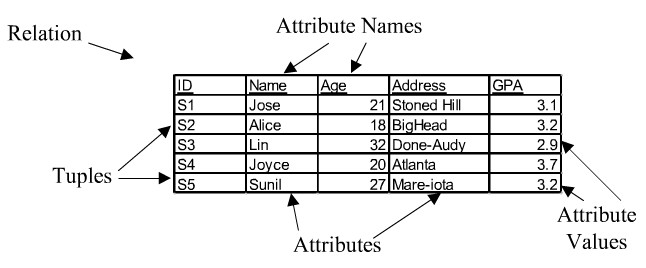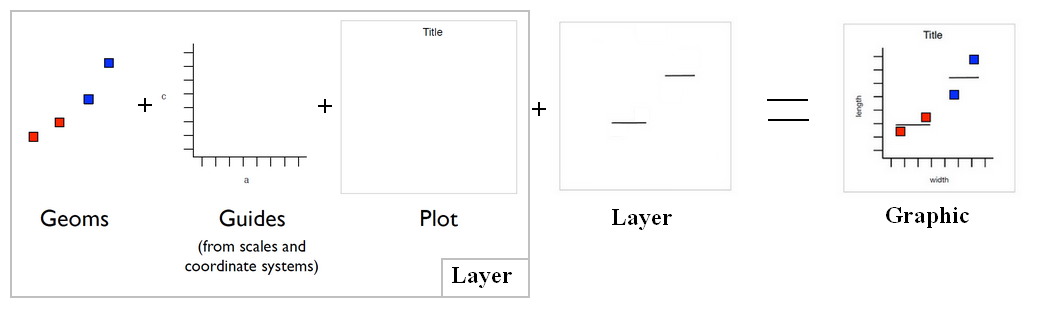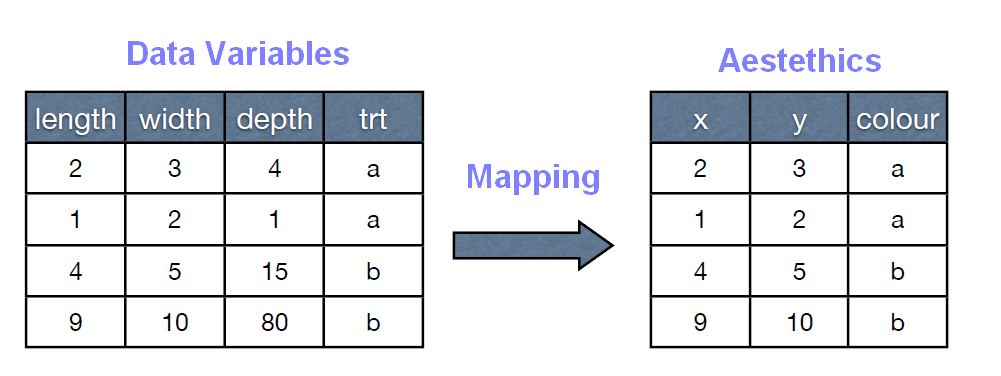# R - Data frame Object

A data frame is a logical implementation of a table in a relational database

A data frame inherits all the property and function of an object.

It has a list of variables of the same number of rows with unique row names.

A matrix-like structure whose columns may be of differing types (numeric, logical, factor and character and so on).

A data frame is a matrix-like structure whose columns may be of differing class (data type)

It's used as the fundamental data structure by most of R's modeling software.

A matrix implementation (array of 2 dimension) also exists

The data frame share many of the properties of matrices and lists.

They can be seen as list where every element of the list has the same length.

## Creation

### Constructor

``````data.frame(...,
row.names = NULL,
check.rows = FALSE,
check.names = TRUE,
stringsAsFactors = default.stringsAsFactors()
)```
```

where:

• … is a list of object that have the same number of rows.
• rownames is a single integer or character string specifying a column to be used as row names, or a character or integer vector giving the row names for the data frame.
• checkrows checks the rows for consistency of length and names if true.

## Persistence

### Import (By reading a file)

````read.table()`
```
• file with a table format and with comma separator (R - Csv)
````read.csv()`
```

Or from the R Studio GUI:

### Export

To clipboard with tabulation which can be paste in Excel

``````writeToClipboard <- function(x,row.names=FALSE,col.names=TRUE,...) {
write.table(x,"clipboard",sep="\t",row.names=row.names,col.names=col.names,...)
}
writeToClipboard(data_frame)```
```

## Construction Example

### Simple

``````colA=c(8,3,6,5,5)
colB=c("Nico","Klaas","Santa","Klaus","Piet")
colC=1:5
df = data.frame(colA,colB,colC)
df```
```
``````colA  colB colC
1    8  Nico    1
2    3 Klaas    2
3    6 Santa    3
4    5 Klaus    4
5    5  Piet    5
```
```

### row.names

By default, if the arguments are all named and simple objects (not lists, matrices of data frames) then the argument names give the column names.

• the rows names are defined by the column B.
````data.frame(colA,colB,colC,row.names=colB)`
```
``````colA  colB colC
Nico     8  Nico    1
Klaas    3 Klaas    2
Santa    6 Santa    3
Klaus    5 Klaus    4
Piet     5  Piet    5
```
```
• the rows names are defined by letters
````data.frame(colA,colB,colC,row.names=letters[1:5])`
```
``````colA  colB colC
a    8  Nico    1
b    3 Klaas    2
c    6 Santa    3
d    5 Klaus    4
e    5  Piet    5
```
```

### check.rows

check.rows will check the names of the rows when two matrix-like structure are given as argument.

``````df1 = data.frame(A=1:2,B=2:1, row.names=letters[1:2])
> df1```
```
``````A B
a 1 2
b 2 1
```
```
``````> df2 = df1[2:1,]
> df2```
```
``````A B
b 2 1
a 1 2
```
```
````data.frame(df1,df2,check.rows=TRUE)`
```
``````Error in data.row.names(row.names, rowsi, i) :
mismatch of row names in arguments of 'data.frame', item 2
```
```

because a,b is not b,a

### check.names

Duplicate column names are allowed, but you need to use check.names = FALSE

## Transformation

### Selection, Modification

Example:

• Select all records with a success_flg equal to 3
````res[res\$SUCCESS_FLG==3,]`
```

``````data_frame\$newColName <- a.vector
data_frame[, "newColName"] <- a.vector
data_frame["newColName"] <- a.vector```
```

### Apply a function

• lapply: Apply a Function over a List or Vector
• by:Apply a Function to a Data Frame Split by Factors

## How to

### Get the number of rows and columns

``````# Number of rows
> nrow(df)
 5
>
> # Number of columns
> ncol(df)
 3```
```

### Check the attributes

``````> attributes(df)
\$names
 "colA" "colB" "colC"

\$row.names
 1 2 3 4 5

\$class
 "data.frame"```
```

### Get the value of a cell

• With indexing:
``````> df[2,1]
 2
> df[1,2]
 Nico
Levels: Klaas Klaus Nico Piet Santa```
```
• With row and column name:
``````> df2["d","colA"]
 5```
```

data.matrix()

### Get the number of rows and columns

``````> df <- data.frame(A=1:2,B=1:2,C=letters[1:2])
> nrow(df)
 2
> ncol(df)
 3```
```

### See the header and the tail

The first two lines:

````head(df,2)`
```

The last two lines:

````tail(df,2)`
```

### Detached the variables name

attach() allows a user to access the variables name (columns) of a data.frame directly.

## Documentation / Reference

Discover More(Relation|Table) - Tabular data

A Relation is a logical data structure composed of tuple (row) attribute (column, field) The following data structure are a relation: a table, a materialized view (query) (store data) a query,...GGplot - Stat - (Statistical transformation|Statistic)

The Statistical transformation (stat). Multiple layers, statistical transformation. It's often useful to transform your data before plotting, and that's what statistical transformations do. Every...Ggplot - (aes|aesthetic) (plot parameter definition)

Aesthetic are plots parameters that are given by the data. For parameters that are not related to the data, see Such as: coordinate x and y. Color: color: the bar outline, fill: interior colouring...ORE - Snippet

ore snippet Connect to the database. Load Data Frame to table Create IRIS_TABLE_N that does not contain SPECIES, the nonnumeric column: The sample null.R is the only sample that does...R - (Object|Variable|Symbol)

R provides a number of specialized objects. They are created (instantiated), used and referenced through variable (known as symbol). When you read the term object in the documentation, you can interchange...R - Class

A class is just an attribute of an object. You can remove the attribute with the function . The class with then become the type A list can have different class. unclass returns (a copy of) its...R - Data Table

A data table is an enhanced data.frame. data.tables (and data.frames) are internally lists as well, but with all its columns of equal length and with a class attribute. data.table function ...R - Database (JDBC, )

It will return a Standard: DBI: Database Interface Definition JDBC: RJDBC ...R - Dplyr (Data Frame Operations)

Dplyr aims to provide a function for each basic verb of data manipulation: filter() to select cases based on their values. arrange() to reorder the cases. select() and rename() to select variables...R - List

The list in R may contain elements of the different class (just like a data frame) class a vector (1 dimension) or a matrix (2 dimensions) data.tables and data.frames are internally lists with all...